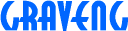2.1 Pushing an object

When an object with mass is moving, that it has kinetic energy is well known. Energy quantity can be expressed in two parameters of the mass and the speed. Generally definition of energy is difficult, though the quantity can be measured easily.

Assume that ball 'A' like billiards with mass 'M' at speed of 'V' collides with center of other standstill ball 'B' as the same peculiarity as ball 'A'.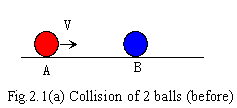I express that ball 'A' has Energy of Motion be in proportion to 'MV'. Before collision, there has zero energy with ball 'B', we don't consider like rest energy, and disregard small loss energy like heat and influence of its rotation in this chapter. After collision, ball 'A' becomes to be at rest, and ball 'B' is moving at velocity of 'V'.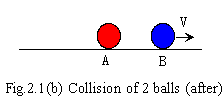It can consider that the energy of motion with ball 'A' transfer to ball 'B'. Energy is movable like this. The whole of energy is the same as before and after This is an instance which most basic and important of being called law of energy conservation. It is hard to measure energy when pushing a wall being at rest by stick, but we can know the energy quantity by pushing a ball with the same force.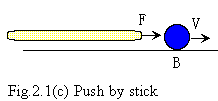Doing like this, even if directly operated energy quantity is unknown, the energy can be known by measuring the speed of the object which energy is added to. Complex operated energy is convertible into simple case.

Incidentally, we have been using quantity of velocity with no particular consciousness, unexpectedly it could not be easy to understand. Velocity is considered as relative quantity in physics. If we suppose so, energy which is function of the velocity would be also relative quantity. By these thought, we will be confronted with a difficult problem right now. Suppose that look at a station from moving train at speed of 'V', the station (universe?) is moving to contrary direction at 'V'. There must be existing enormous energy of motion which consists of the station mass and velocity of 'V'. Could we extract this energy? There is not exist reality, it seem to fall often into unrealistic world under mathematical expression in physics. It is considered not to perform role of physics to express phenomenon to mathematical correctly. In existent physics, is there a way to express that train is moving, but station? How do we confirm it? Confirming it is not too difficult. Reducing train velocity to zero relative velocity between train and station, we can measure released energy quantity. We could confirm that the energy is proportion of train velocity 'V' and train mass. We could consider that train had been moving, but station by this measuring. However, observing from coordinates which moving with train as zero relative velocity, it must be a fact that moving is station, train is rest. Which is moving truly? A person who had tried to understand physics from basic probably become aware of the question that oldest unsolved spatial problem since Newton. Absolute space of Newton had been denied, velocity of object and motion have been considered as relative quantity in general physics. However you would probably find out that these thought is contradictory the experiment. If not exist non-relative velocity conception, we would become not to be able to express phenomenon to mathematical.

We are necessary for reviewing about concept of velocity and space. Next chapter, we will discover conception of absolutely velocity ( not absolute velocity ).

Updated 29/Mar/1997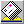redsky@graveng.jp